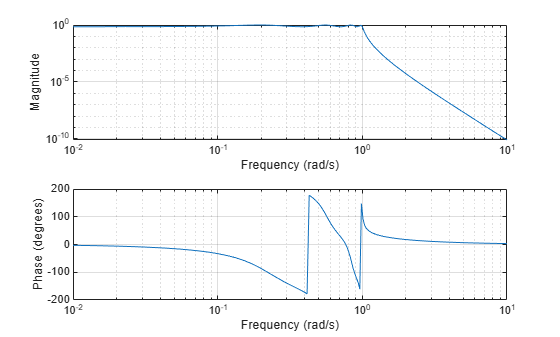lp2lp

Change cutoff frequency for lowpass analog filter

Description

example

[bt,at] = lp2lp(b,a,Wo) transforms an analog lowpass filter prototype given by polynomial coefficients (specified by row vectors b and a) into a lowpass filter with cutoff angular frequency Wo. The input system must be an analog filter prototype.

[At,Bt,Ct,Dt] = lp2lp(A,B,C,D,Wo) converts the continuous-time state-space lowpass filter prototype (specified by matrices A, B, C, and D) to a lowpass filter with cutoff angular frequency Wo. The input system must be an analog filter prototype.

Examples

collapse all

Design an 8th-order Chebyshev Type I analog lowpass filter prototype with 3 dB of ripple in the passband.

[z,p,k] = cheb1ap(8,3);

Convert the prototype to transfer function form and display its magnitude and frequency responses.

[b,a] = zp2tf(z,p,k);
freqs(b,a)Transform the prototype to a lowpass filter with a cutoff frequency of 30 Hz. Specify the cutoff frequency in rad/s. Display the magnitude and frequency responses of the transformed filter.

Wo = 2*pi*30;

[bt,at] = lp2lp(b,a,Wo);
freqs(bt,at)Input Arguments

collapse all

Prototype numerator and denominator coefficients, specified as row vectors. b and a specify the coefficients of the numerator and denominator of the prototype in descending powers of s:

$\frac{B\left(s\right)}{A\left(s\right)}=\frac{b\left(1\right){s}^{n}+\cdots +b\left(n\right)s+b\left(n+1\right)}{a\left(1\right){s}^{m}+\cdots +a\left(m\right)s+a\left(m+1\right)}$

Data Types: single | double

Prototype state-space representation, specified as matrices. The state-space matrices relate the state vector x, the input u, and the output y through

$\begin{array}{l}\stackrel{˙}{x}=Ax+Bu\\ y=Cx+Du\end{array}$

Data Types: single | double

Cutoff angular frequency, specified as a scalar. Express Wo in units of rad/s.

Data Types: single | double

Output Arguments

collapse all

Transformed numerator and denominator coefficients, returned as row vectors.

Transformed state-space representation, returned as matrices.

Algorithms

lp2lp transforms an analog lowpass filter prototype with a cutoff angular frequency of 1 rad/s into a lowpass filter with any specified cutoff angular frequency. The transformation is one step in the digital filter design process for the butter, cheby1, cheby2, and ellip functions.

lp2lp is a highly accurate state-space formulation of the classic analog filter frequency transformation. If a lowpass filter has cutoff angular frequency ω0, the standard s-domain transformation is

$s=p/{\omega }_{0}$

The state-space version of this transformation is

$At={\omega }_{0}\cdot A$

$Bt={\omega }_{0}\cdot B$

$Ct=C$

$Dt=D$

The lp2lp function can perform the transformation on two different linear system representations: transfer function form and state-space form. See lp2bp for a derivation of the bandpass version of this transformation.